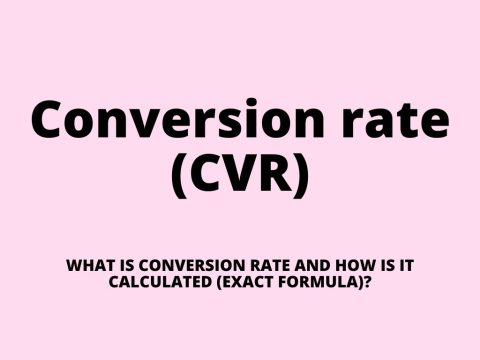# bullet points#### Conversion rate (CVR) – what is conversion rate and how is CVR calculated (exact formula)?

A conversion rate is a marketing metric that quantifies the proportion of instances when a user successfully completed a desired action, usually expressed as a percentage. What is a conversion ...

A conversion rate is a marketing metric that quantifies the proportion of instances when a user successfully completed a desired action, usually expressed as a percentage. What is a conversion Read article

#### Which of the following is NOT a best practice when creating knowledge base articles?

Below is the answer and explanation for which of the following is not a best practice when creating knowledge base articles? Which of the following is not a best practice when creating knowledge base articles? Use questions ...

Below is the answer and explanation for which of the following is not a best practice when creating knowledge base articles? Which of the following is not a best practice when creating knowledge base articles? Use questions Read article

#### How to write/type inverse bullet symbols/emojis on keyboard- Alt + 8

In typography, an inverse bullet symbol or inverse bullet point, ◘, is a glyph or typographical symbol used to introduce items in a list. For example: ◘ Point 1 ◘ Point 2 ◘ Point 3 The bullet symbol may take ...

In typography, an inverse bullet symbol or inverse bullet point, ◘, is a glyph or typographical symbol used to introduce items in a list. For example: ◘ Point 1 ◘ Point 2 ◘ Point 3 The bullet symbol may take Read article